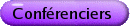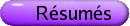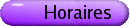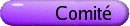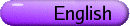Théorie des jeux et des nombres
Org: Richard Nowakowski (Dalhousie), Bill Sands (Calgary), Hugh Williams (Calgary) et Robert Woodrow (Calgary)
[PDF]

MIKE BENNETT, University of British Columbia, Vancouver, BC
The prime factorization of binomial coefficients
[PDF]

Let us suppose that n and k are positive integers with n ³ 2k, and factor the binomial coefficient \binomnk = U ·V, where U is comprised of those primes not exceeding k and V contains those primes exceeding k. Then an old theorem of Ecklund, Eggleton, Erdös and Selfridge asserts that V > U, with at most finitely many exceptions (all of which are conjectured to be known). We will take a rather different approach to this problem than that of Ecklund, Eggleton, Erdös and Selfridge, enabling us to resolve their conjecture in two of the three remaining cases.

This is joint work with M. Filaseta and O. Trifonov.

ELWYN BERLEKAMP, University of California, 2039 Shattuck Ave, Berkeley, CA 94704
Yellow-Brown Hackenbush
[PDF]

Yellow-Brown Hackenbush is a game played on a sum of strings whose branches are colored yellow or brown. In its "restricted" form, one player, named Left, at her turn, picks a bichromatic string and removes its highest yeLLow branch. Right, at his turn, picks a bichromatic string and removes its highest bRown branch. As in the well-known game of bLue-Red Hackenbush, all higher branches, being disconnected, also disappear. But in yellow-brown Hackenbush, unlike blue-red Hackenbush, all moves on monochromatic strings are illegal. This makes all values of yellow-brown Hackenbush all-small.

This paper presents an explicit solution of restricted yellow-brown Hackenbush. The values are sums of basic infinitesimals that have appeared in many other games found in Winning Ways and elsewhere.

PETER BORWEIN, Simon Fraser University
Littlewood's 22nd Problem
[PDF]

Littlewood, in his 1968 monograph "Some Problems in Real and Complex Analysis", poses the following research problem, which appears to still be open:

"If the nm are integral and all different, what is the lower bound on the number of real zeros of

 Nå m=1 cos(nmq)?
Possibly N-1, or not much less."

No progress appears to have been made on this in the last half century. Until now!

DAVID BOYD, University of British Columbia, Vancouver, BC
Pisot sequences with periodic rounding rules
[PDF]

The classical Pisot sequence E(a0,a1) is defined by the non-linear recurrence an+2 = N(an+12/an), where a0 < a1 are positive integers and N(x) means round x to the nearest integer. One can define a variety of different sequences by replacing N(x) by U(x) or D(x) where these mean round x up or down to the closest integer, respectively. Here we consider rounding rules which apply the operators U and D in a periodic fashion, e.g. the sequence UUDUD(a0,a1) would start by rounding up for the next two rounds, then down, then up, then down, repeating this indefinitely. One is interested in subset of such sequences which satisfy linear recurrence relations. We show that there is a striking difference between the case in which the rounding rule has minimal period at most 2, and the case in which this period is greater than 2. The results have some applications to questions about classical Pisot sequences.

ANDREW BREMNER, Arizona State University, Tempe, AZ 85287-1804, USA
A problem in right triangles
[PDF]

We investigate the following problem mentioned in Dickson's History, Vol. 2, Chapter 4, on rational right triangles (evidently it was posed in obscure verse in The Ladies Diary for 1728 as Question 133, but seems to be based on a numerical example of Ozanam from 1702):

Find a right triangle each of whose sides exceeds double the area by a square.

We analyze the mathematics behind the problem, and inter alia find an example with smallest possible standard generators for the underlying Pythagorean triangle.

The observation is also made that mathematicians whose names are anagrams of rivers seem to be particularly scarce.

DENIS CHARLES, Microsoft Research
Some applications of the graph of supersingular elliptic curves over a finite field
[PDF]

The graph of supersingular elliptic curves over a finite field connected by isogenies has many applications in computational number theory. In this talk we look at some old (in number theory) and new (in cryptography) applications of these graphs. In particular, we discuss new constructions of secure hash functions and pseudorandom number generators from these graphs.

KARL DILCHER, Dalhousie University, Halifax, Nova Scotia, B3H 3J5
Divisibility properties of certain binomial sums
[PDF]

We study congruence and divisibility properties of a class of combinatorial sums that involve products of powers of two binomial coefficients, and show that there is a close relationship between these sums and the theorem of Wolstenholme. We also establish congruences involving Bernoulli numbers, and finally we prove that under certain conditions the sums are divisible by all primes in specific intervals.

This is joint work with Marc Chamberland.

AVIEZRI FRAENKEL, Weizmann Institute of Science, Rehovot, Israel
Can one perceive the alpine wind of a game?
[PDF]

Nim and chess are both combinatorial games with perfect information and no chance moves. Why is Nim easy and chess hard? There are several mathematical differences between them. Previously we have launched a concentrated attack on each of the differences separately, since this divide-and-conquer approach has a better chance of answering our question than a direct attempt to scale the sheer cliff separating polynomial Nim from Exptime-complete chess. We thus ascended from sea-level Nim towards alpine-heights chess at a moderate gradient, by gradually introducing into Nim more and more complications in a natural order of increasing complexities. What happens at the higher elevations, when we have already introduced cycles and a capture rule, but games are still impartial? We will attempt to show how one can hear and feel the breeze of the crisp alpine wind blowing out of such games. The talk is dedicated to Richard Guy, who is both a leading gamester and a keen member of the Alpine Club of Canada; both of these activities made him 90 years young!

CARL POMERANCE, Dartmouth College, Hanover, NH 03755, USA
Covering congruences
[PDF]

Over 50 years ago, Paul Erdös conjectured that the integers can be covered by a finite collection of residue classes ai mod ni with distinct moduli ni, and with the least modulus arbitrarily large. So far, the record is due to Morikawa in 1984, who has found such a covering system with least modulus 24. This, and similar problems, are discussed extensively in Guy's UPINT. We solve one of these problems, namely, we prove the conjecture of Erdös and Selfridge that in a covering system with large least modulus, the reciprocal sum of the moduli must also be large. In addition, we prove the conjecture of Erdös and Graham that for each K > 1, there is a positive number dK such that if the moduli all come from an interval [N,KN], where N is large, then for any choice of residue classes for these moduli, at least density dK of the integers remain uncovered.

This work is joint with Michael Filaseta, Kevin Ford, Sergei Konyagin, and Gang Yu.

RENATE SCHEIDLER, University of Calgary, Department of Mathematics, 2500 University Drive NW, Calgary, AB T2N 1N4
Units in Cubic Function Fields
[PDF]

An efficient algorithm for computing the fundamental unit, or equivalently, the regulator, of a real quadratic field has so far eluded researchers. Similarly, finding the the fundamental unit(s) of a cubic field remains computationally hard for large field sizes, and the task only becomes messier as the degree of the field extension increases. Interestingly, both the quadratic and the cubic scenario employ different variants of the simple continued fraction algorithm to generate the fundamental unit(s).

The task at hand seems to be just as hard for function fields. The best known methods here are basically extensions of the number field methods, but there are subtle differences which we will explain. In this talk, we focus mainly on unit computation in cubic function fields. We explain how to determine the unit rank of such an extension and how to find a system of fundamental units using an extension of Voronoi's algorithm. One of the main obstacles to efficient unit computation is the huge size of the fundamental units-they are generally exponential in the size of the field-so as a matter of curiosity, we also provide parameterized families of purely cubic function fields with unusually small fundamental units.

AARON SIEGEL, Mathematical Sciences Research Institute
The Misère Mex Mystery
[PDF]

Under the normal play condition on an impartial game, the player who makes the last move wins. Under the misère play condition, whoever makes the last move loses. It was long ago observed that misère games are vastly more difficult than their normal counterparts.

It was also observed that in the case of Nim, there is a curious correspondence. The strategy for misère Nim is: Follow the strategy for normal Nim until your move would leave no heaps of size greater than one. Then play to leave an odd number of heaps of size one.

We will show that this correspondence generalizes to many misère games, including many two- and three-digit octals. For each such game G, the strategy for misère G is: Follow the strategy for normal G as long as the position remains sufficiently rich, in a sense that depends on G. Then pay attention to the fine structure of the misère quotient.

This broad strategic principle manifests itself in certain structural properties of the misère quotient of G, and is tied to deep questions about how the mex rule generalizes to misère play. We will discuss this relationship and raise a number of intriguing conjectures.

This is joint work with Thane Plambeck.

DAVID SINGMASTER, 87 Rodenhurst Road, London, SW4 8AF, UK
17 Camels, 13 Camels, 11 Bridges, 3 Rabbits, Coconuts
[PDF]

Despite its age and simplicity, recreational mathematics constantly presents topics for historical and mathematical investigation. The problem of the 17 camels is often claimed to be ancient, but the earliest known example is from 1872. The 13 camels variant is only known from one author, in 1971. Mathematical investigation determines all possible forms of these problems for small families and finds some extended pseudo-solutions.

I will then give some brief descriptions of work on the 11 [sic!] bridges of Konigsberg, the puzzle of the Three Rabbits [How do you draw three rabbits, each with two ears, but using only three ears in all?], the Monkey and the Coconuts as done by Lewis Carroll, etc.

ALF VAN DER POORTEN, ceNTRe for Number Theory Research, Sydney
Curious cubes and self-similar sums of squares
[PDF]

I will take mild issue with Hardy's dismissive remark:

"There are just four numbers (after 1) which are the sums of the cubes of their digits, viz. 153 = 13+53+33, 370 = 33+73+03, 371 = 33+73+13, and 407 = 43+03+73. This is an odd fact, very suitable for puzzle columns and likely to amuse amateurs, but there is nothing in it which appeals much to a mathematician. The proof is neither difficult nor interesting-merely a little tiresome. The theorem is not serious; and it is plain that one reason (though perhaps not the most important) is the extreme speciality of both the enunciation and the proof, which is not capable of any significant generalization."

In retaliation I nominate 13+53+33 = 153, 163+503+333 = 165033, 1663+5003+3333 = 166500333, 16663+50003+33333 = 16665000333, ..., and turning to squares, 122+332 = 1233, 882+332 = 8833, .... Of course that last pair of examples is mathematically far more interesting, and I concentrate on its generalisation by reporting on work done some years ago jointly with Kurt Thomsen and Mark Wiebe, at the time undergraduate students at the University of Manitoba.

STAN WAGON, Macalester College, St. Paul, MN  55105, USA
The postage-stamp problem: an application of geometry to number theory
[PDF]

Given a set A of finitely many positive integers (the denominations), the Frobenius problem comes in two flavors:

(1) determining, for a given target M, whether some nonnegative combination of the denominations sums to M, and if so, finding a representation;
(2) computing the Frobenius number f(A), which is the largest M that is not representable.

For example, if A = 6, 9, 20, then f(A) = 43. The main approaches to (2) have used graph theory and have been limited to denominations no greater than about 10 million. We will show how a detailed study of a certain geometrical polyhedron leads to a fast solution that works with no restriction on the size of the denominations.

Joint work with David Einstein, Daniel Lichtblau, and Adam Strzebonski.

GARY WALSH, University of Ottawa, 585 King Edward St., Ottawa, Ontario K1N 6N5
Don't try to solve these Diophantine equations
[PDF]

Although the problem of determining all integer points on an elliptic curve can readily be solved by any number of math packages nowadays, it is often the case that such problems resist elementary approaches. We will discuss just such a problem, due to Martin Gardner, along with a related family of elliptic curves (and related Thue equations) that continue to resist solution by any known method, elementary or not.

DAVID WOLFE, Gustavus Adolphus College, Minnesota
Introducing New Games
[PDF]

New games invented (or discovered) in the last few years include Toppling Dominoes, Shove (and Push), and Maze (and Maize). These games have simple, playable rules and include our favorite game values such as numbers, ups and stars, but the values can appear in surprising ways.

Work is in conjunction with Michael Albert and Richard Nowakowski.